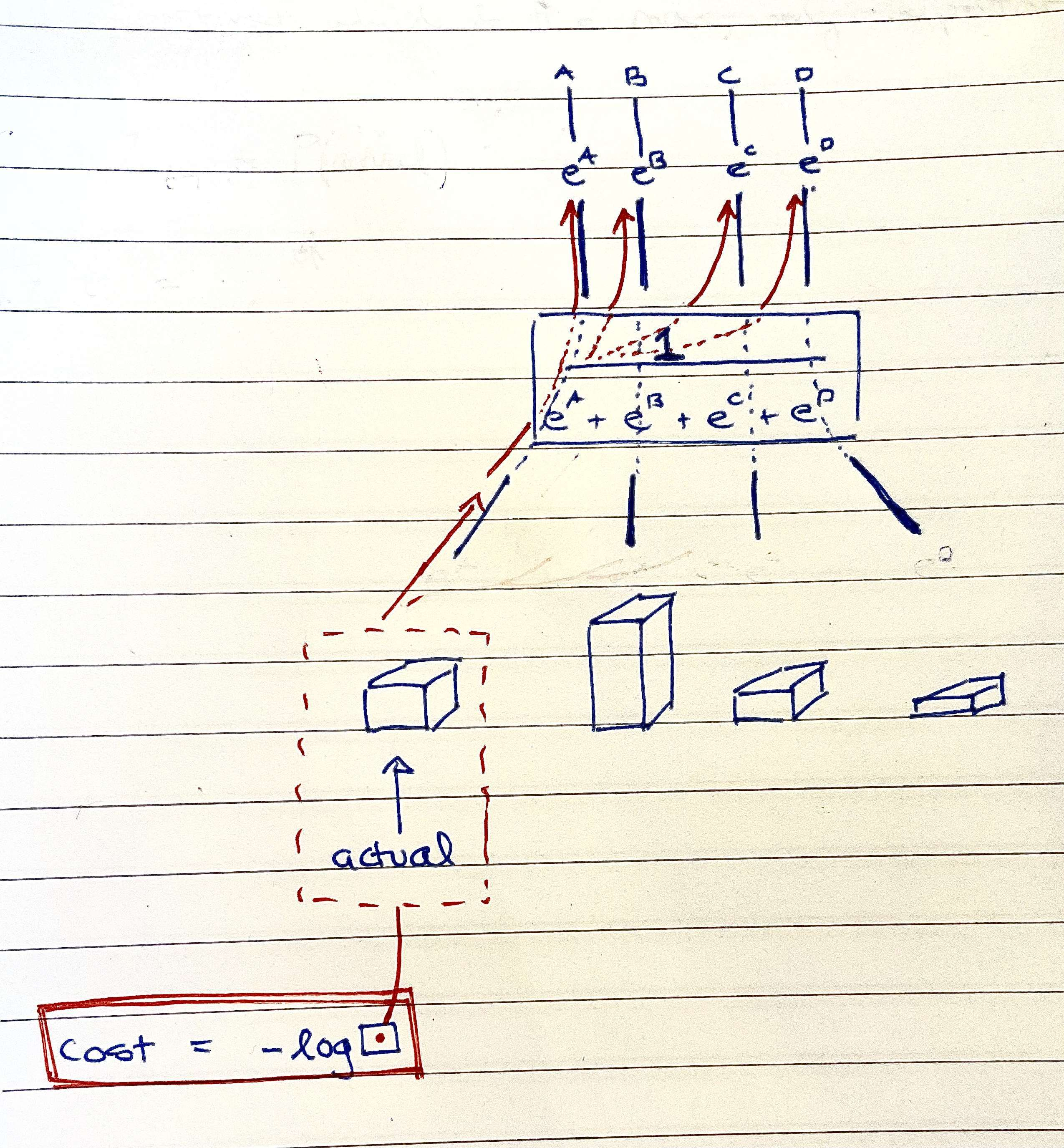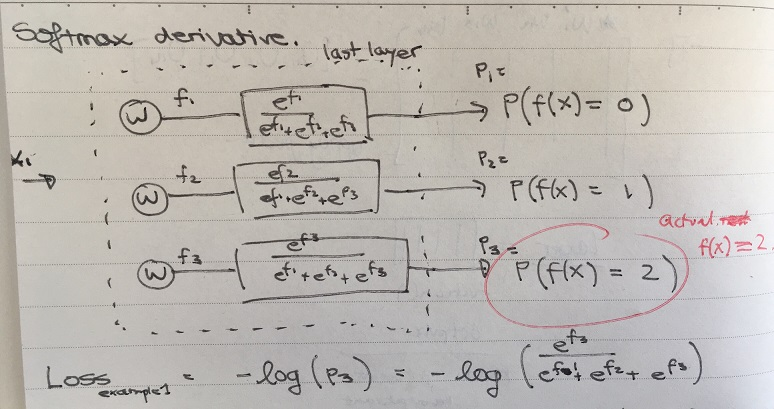Show Question
What is the derivative of negative log MLE (MLE used as a negative cost) when the variables are passed through softmax activations?

The derivative of the cost function is needed for back-propagation.

When the output layer uses a MLE/cross-entropy cost and softmax activation, the derivative of the cost function combined with the activation function simplifies to a very simple expression. This note covers this computation.

Consider the last layer of a neural network shown below:For the given training example $$x_1$$, the correct category is 2 (out of all categories 0,1 and 2). Only the output corresponding to category 2 is used in the calculation of the loss contributed by the example. The loss is given by:

$Loss_{ex_1} = -ln(p_3) = -ln(\frac{e^{f_3}}{e^{f_1} + e^{f_2} + e^{f_3}})$

While only the probability for the correct category is used to calculate the loss for the example, the other outputs, $$p_1$$ and $$p_2$$ still affect the loss, as $$p_3$$ depends on these values (all sum to 1).

The partial derivatives will be:

\begin{align*} \frac{\partial L}{\partial f_1} &= p_1 \\ \frac{\partial L}{\partial f_2} &= p_2 \\ \frac{\partial L}{\partial f_3} &= -(1-p_3) = p_3 - 1\end{align*}

So if we have output probabilities [0.1, 0.3, 0.6], then the derivative of the loss with respect to last layer's output before applying the softmax activation will be [0.1, 0.3, -0.4].

### Derivations

Formulating the derivative of loss wrt different variables is simplified if the log and exponential are canceled at the beginning:

\begin{align*} Loss_{ex_1} = -ln(p_3) &= -ln(\frac{e^{f_3}}{e^{f_1} + e^{f_2} + e^{f_3}}) \\ &= ln(\frac{e^{f_1} + e^{f_2} + e^{f_3}}{e^{f_3}}) \\ &= ln(e^{f_1} + e^{f_2} + e^{f_3}) - ln(e^{f_3}) \\ &= ln(e^{f_1} + e^{f_2} + e^{f_3}) - f_3 \end{align*}

From here, the partial derivates are obvious. For example:

\begin{align*} \frac{\partial L}{\partial f_1} &= \frac{\partial}{\partial f_1}(ln(e^{f_1} + e^{f_2} + e^{f_3}) - f_3) \\ &= \frac{\partial}{\partial x}(ln(x)) \frac{\partial}{\partial f_1}(e^{f_1} + e^{f_2} + e^{f_3}) \\ &= \frac{1}{x} e^{f_1} \\ &= \frac{e^{f_1}}{e^{f_1} + e^{f_2} + e^{f_3}} \\ &= p_1 \end{align*}

The below image is part of a separate card, but I'm including it here, as it is quite relevant.Old image: